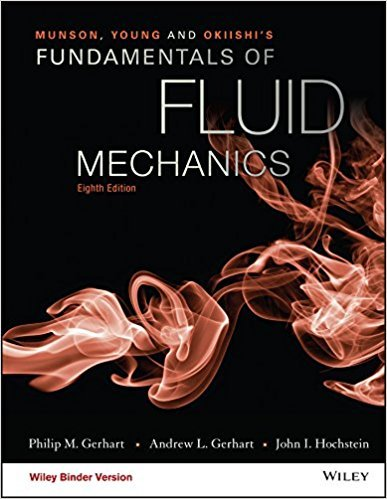×
×

# Air flows steadily between two cross sections in a long,straight section ofISBN: 9781119080701 456

## Solution for problem 5.9 Chapter 5.1.2

Fundamentals of Fluid Mechanics | 8th Edition

• Textbook Solutions
• 2901 Step-by-step solutions solved by professors and subject experts
• Get 24/7 help from StudySoup virtual teaching assistantsFundamentals of Fluid Mechanics | 8th Edition

4 5 1 307 Reviews
30
4
Problem 5.9

Air flows steadily between two cross sections in a long,straight section of 0.1-m-inside-diameter pipe. The static temperatureand pressure at each section are indicated in Fig. P5.9. If theaverage air velocity at section (1) is 205 m/s, determine the averageair velocity at section (2).

Step-by-Step Solution:
Step 1 of 3

Evolution of skin color  Skin color—one of the most conspicuous signs of human variation  Results from combination of evolutionary selection pressure o Especially differences in exposure to UVR  UVR has 2 adverse effects o Causes skin cancer o Breaks down folic acid needed for normal cell division, fertility, and fetal development  UVR has...

Step 2 of 3

Step 3 of 3

##### ISBN: 9781119080701

Since the solution to 5.9 from 5.1.2 chapter was answered, more than 265 students have viewed the full step-by-step answer. The answer to “Air flows steadily between two cross sections in a long,straight section of 0.1-m-inside-diameter pipe. The static temperatureand pressure at each section are indicated in Fig. P5.9. If theaverage air velocity at section (1) is 205 m/s, determine the averageair velocity at section (2).” is broken down into a number of easy to follow steps, and 43 words. Fundamentals of Fluid Mechanics was written by and is associated to the ISBN: 9781119080701. This full solution covers the following key subjects: . This expansive textbook survival guide covers 112 chapters, and 1357 solutions. This textbook survival guide was created for the textbook: Fundamentals of Fluid Mechanics, edition: 8. The full step-by-step solution to problem: 5.9 from chapter: 5.1.2 was answered by , our top Science solution expert on 03/16/18, 03:21PM.

Unlock Textbook Solution